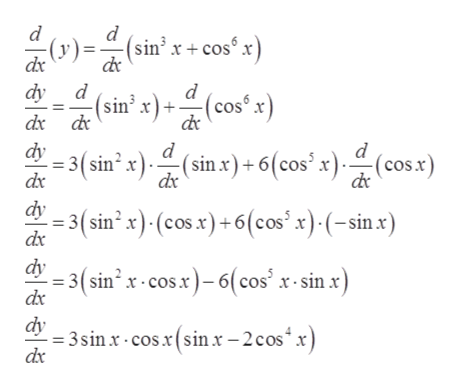Question
8 views

find dy/dx where y=sin^3x+cos^6x

check_circle

Step 1

Given:

Step 2

Formula used:

Step 3

Hence solving the given question:&n...help_outlineImage Transcriptionclosed d (y) dx (sin3 x +cos° x dy d dx d dy - (sin' x)() (cos x d ' x) (cos x) (sinx)+6(cos 3( sin2 x dx dx 3(sin2 x) (cosx) + 6 (cos" x) - (-sin x) dy (sin x-cos.x)-6(cos x-sin x) dx dy 3sin x cosx(sin.x -2 cos x dx fullscreen

### Want to see the full answer?

See Solution

#### Want to see this answer and more?

Solutions are written by subject experts who are available 24/7. Questions are typically answered within 1 hour.*

See Solution
*Response times may vary by subject and question.
Tagged in

### Derivative